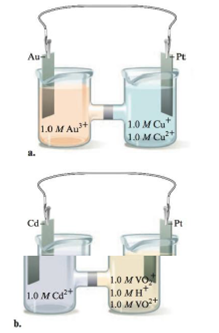Chapter 18, Problem 45E

Chapter
Section
Textbook Problem

# Consider the following galvanic cells:For each galvanic cell, give the balanced cell equation and determine. Standard reduction potentials are found in Table 17-1.

(a)

Interpretation Introduction

Interpretation:

The set up of two galvanic cells is given. The balanced cell equation and the value of E° for each cell is to be stated.

Concept introduction:

The species at the anode undergoes oxidation while the species at the cathode undergoes reduction. The species with higher reduction potential undergoes reduction while the species with lower reduction potential undergoes oxidation.

To determine: The balanced cell equation and E° for the given galvanic cell.

Explanation

The balanced cell equation is,

Au3+(aq)+3Cu+(aq)Au(s)+3Cu2+(aq)

The value of E°cell is 1.34V_ .

The reaction taking place at cathode is,

Au3+(aq)+3eAu(s)E°red=+1.50V

The reaction taking place at anode is,

Cu+(aq)Cu2+(aq)+eE°ox=0

(b)

Interpretation Introduction

Interpretation:

The set up of two galvanic cells is given. The balanced cell equation and the value of E° for each cell is to be stated.

Concept introduction:

The species at the anode undergoes oxidation while the species at the cathode undergoes reduction. The species with higher reduction potential undergoes reduction while the species with lower reduction potential undergoes oxidation.

To determine: The balanced cell equation and E° for the given galvanic cell.

### Still sussing out bartleby?

Check out a sample textbook solution.

See a sample solution

#### The Solution to Your Study Problems

Bartleby provides explanations to thousands of textbook problems written by our experts, many with advanced degrees!

Get Started

#### Find more solutions based on key concepts